# ResearchRasmus Bartholin

In the 17th century, Denmark fostered two mathematicians of international renown: Rasmus Bartholin, who contributed to the second Latin edition of Descartes’ La Géométrie and Georg Mohr, who proved that one can perform all the Euclidean constructions with circle alone (i.e. without straightedge). The former was a professor at Copenhagen University, the second was not.

During the 18th and the beginning of the 19th century Danish mathematics was rather provincial but around 1870 the two childhood friends and professors of mathematics H.G. Zeuthen and Julius Petersen lifted it up to an internationally recognized level, Zeuthen with his works on enumerative geometry and history of mathematics, Petersen with contributions to graph theory, algebra and geometric constructions.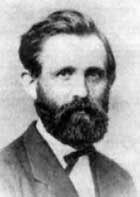Hieronymus Georg Zeuthen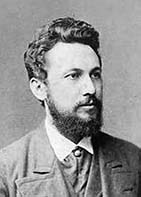Julius Petersen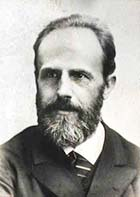Thorvald Nicolai Thiele

At about the same time N. Thiele and J.P. Gram made notable contributions to Statistics. Petersen’s and Zeuthen’s interests in geometry were continued by J. Hjelmslev and the topologist P. Heegaard but from 1930 mathematical analysis became the central discipline at the Department of Mathematics with the works of Niels Nielsen, N.E. Nørlund and in particular Harald Bohr who created the theory of almost periodic functions, and his student Børge Jessen. The latter two were the first heads of the new department.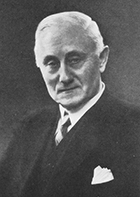J. Hjelmslev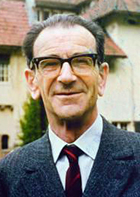Werner Fenchel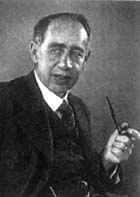Harald Bohr

Also, W. Fenchel’s and Bonnesen’s contributions to convexity theory became internationally recognized.

Mathematical analysis remained a central discipline during the second half of the 20th century with important contributions to operator algebra, potential theory, orthogonal polynomials, partial differential equations and harmonic analysis, but also algebra was strengthened.

During the end of the 20th century and the beginning of the new millennium mathematical physics, topology, algebraic geometry and number theory have become important fields of research at the department.

The research at the Department of Mathematical Statistics was, as the name indicates, characterized by a high level of mathematical abstraction. This has remained the case after the merger, but recently biostatistics (and –mathematics) has become a central area of interest.

Where the research at the Department of Mathematics was almost exclusively pure mathematics, the three mergers and the inclusion of mathematical economy have resulted in a more even distribution of the research between pure and applied mathematics at the Department of Mathematical Sciences.

> Next: Funding